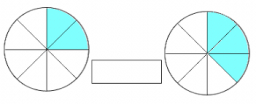Unknown number 32

What number am I? I am greater than 10 but less than 20. My 1/3 is equal to my 1/5 plus 2.

x =  15

Step-by-step explanation:Did you find an error or inaccuracy? Feel free to write us. Thank you!Tips to related online calculators
Need help to calculate sum, simplify or multiply fractions? Try our fraction calculator.
Do you have a linear equation or system of equations and looking for its solution? Or do you have a quadratic equation?